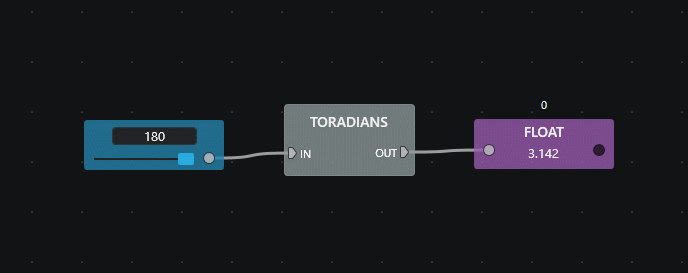# Math¶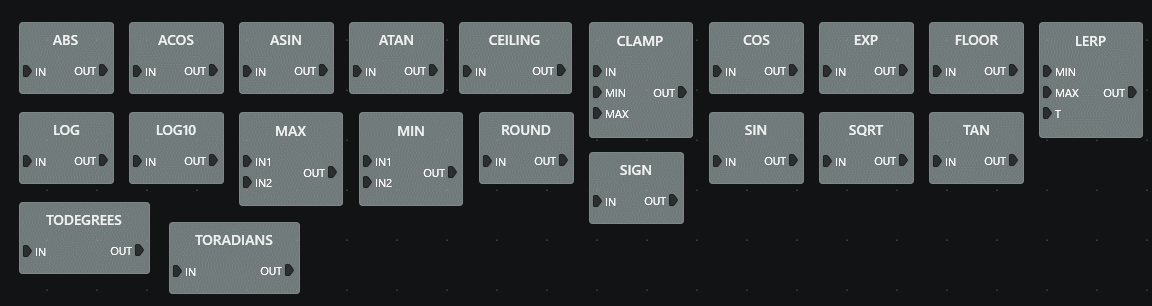## ABS¶

Calculates the absolute value of the input number.## ACOS¶

Calculates the angle whose cosine is the input number. Domain of input for a real result is -1 to 1. Output range is 0 to Π (0 to 180°).## ASIN¶

Calculates the angle whose sine is the input number. Domain of input for a real result is -1 to 1. Output range is -Π/2 to Π/2 (-90° to 90°).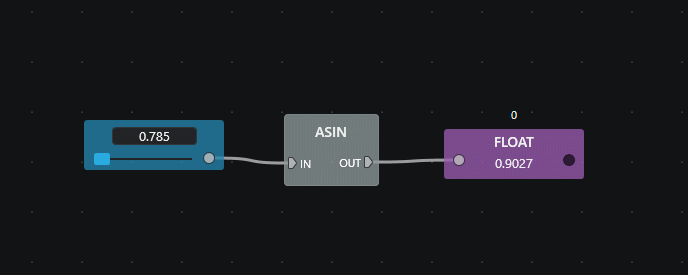## ATAN¶

Calculates the angle whose tangent is the input number. Can be used with all real numbers. Output range is -Π/2 to Π/2 (-90° to 90°).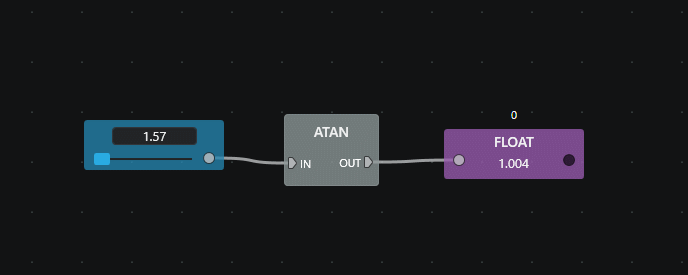## CEILING¶

Calculates the smallest integer greater to or equal to the input number.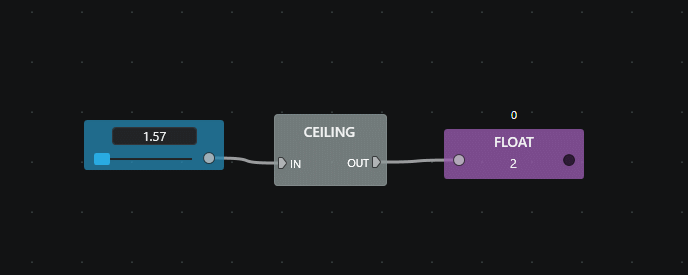## CLAMP¶

Clamps the input number between a minimum and maximum value.## COS¶

Calculates the cosine of the input angle (in radians).## EXP¶

Calculates e raised to the input power.## FLOOR¶

Calculates the largest integer smaller to or equal to the input number.## LERP¶

Performs a linear interpolation between two values. The input value T ranges from 0 to 1.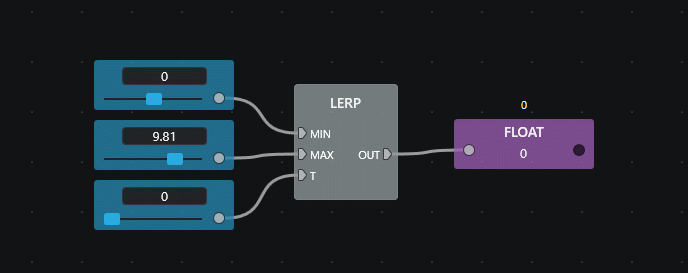## LOG¶

Calculates the base e logarithm of the input value.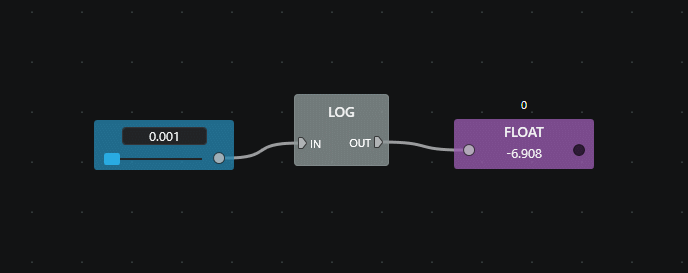## LOG10¶

Calculates the base 10 logarithm of the input value.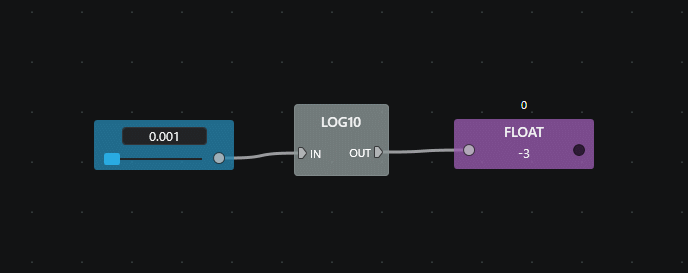## MAX¶

Calculates the larger of two input numbers.## MIN¶

Calculates the smaller of two input numbers.## ROUND¶

Rounds the input value to the nearest integer.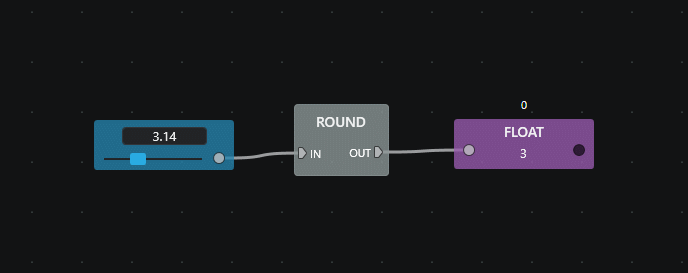## SIGN¶

Calculates the sign of the input number.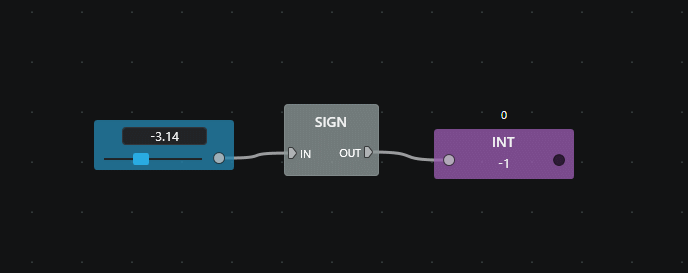## SIN¶

Calculates the sine of the input angle (in radians).## SQRT¶

Calculates the square root of the input number## TAN¶

Calculates the tangent of the input angle (in radians).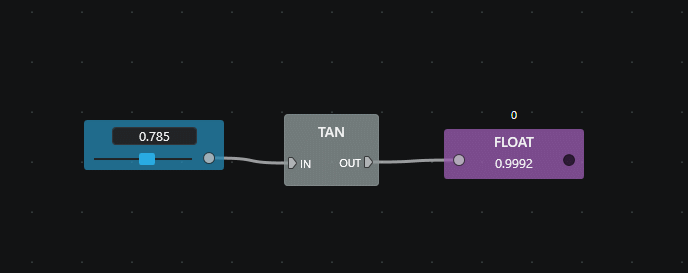## TODEGREES¶

Converts the input value from radians to degrees.